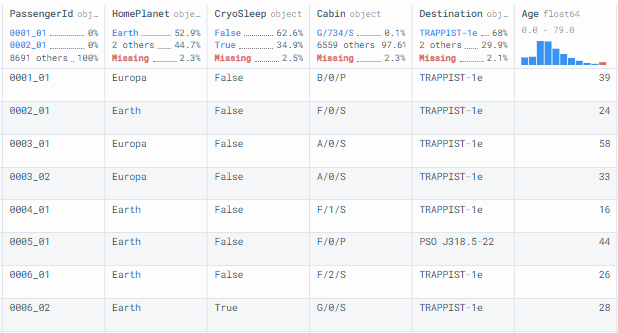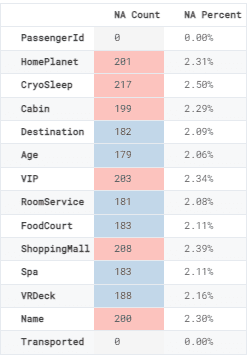# Using Scikit-learn’s Imputer

Learn about Scikit-learn’s SimpleImputer, IterativeImputer, KNNImputer, and machine learning pipelines.Puzzle shape photo created by freepik

# What is an Imputer?

If you have some missing values in your dataset, you can drop the missing values row or even column. This method is highly discouraged as it reduces the size of data, and the data analysis can be skewed from the ground truth. Instead, we should use machine learning algorithms that are not affected by missing values or use imputers to fill in the missing information.

The imputer is an estimator used to fill the missing values in datasets. For numerical values, it uses mean, median, and constant. For categorical values, it uses the most frequently used and constant value. You can also train your model to predict the missing labels.

In the tutorial, we will learn about Scikit-learn’s SimpleImputer, IterativeImputer, and KNNImputer. We will also create a pipeline to impute categorical and numerical features and feed them into a machine learning model.

# How to use Scikit-learn's Imputer

The scikit-learn’s imputation functions provide us with an easy-to-fill option with few lines of code. We can integrate these imputers and create pipelines to reproduce results and improve machine learning development processes.

## Getting Started

We will be using the Deepnote environment, which is similar to Jupyter Notebook but on the cloud.

To download and unzip data from Kaggle. You have to install the Kaggle Python package and download the spaceship-titanic dataset using API. Finally, unzip the data into the dataset folder.

```%%capture
!pip install kaggle
!unzip -d ./dataset spaceship-titanic
```

Next, we will import the required Python Packages for data ingestion, imputation, and creating transformation pipelines.

```import numpy as np
import pandas as pd
from sklearn.impute import SimpleImputer
from sklearn.experimental import enable_iterative_imputer
from sklearn.impute import IterativeImputer,KNNImputer
from sklearn.pipeline import FeatureUnion,make_pipeline,Pipeline
from sklearn.compose import ColumnTransformer
```

The Spaceship Titanic dataset is part of Kaggle’s getting started prediction competition. It consists of train, test, and submission CSV files. We will be using train.csv, which contains passenger information on space ships.

The pandas read_csv() function reads the train.csv and then displays the dataframe.

```df = pd.read_csv("dataset/train.csv")

df
```## Data Analysis

In this section, we will explore columns with missing values, but first, we need to check the shape of the dataset. It has 8693 rows and 14 columns.

```df.shape

>>> (8693, 14)
```

We will now display missing value count and percentage based on columns. To display it in a dataframe, we will create a new dataframe of missing values and apply style gradients to the NA Count column.

```NA = pd.DataFrame(data=[df.isna().sum().tolist(), ["{:.2f}".format(i)+'%' \
for i in (df.isna().sum()/df.shape*100).tolist()]],
columns=df.columns, index=['NA Count', 'NA Percent']).transpose()

```Except for PassengerID and Transported, there are missing values in every column.

## Numerical Imputation

We will use the information in missing columns and divide it into categorical and numerical columns. We will treat them differently.

For numerical Imputation, we will select the Age column and display the number missing value. It will help us validate the before and after results.

```all_col = df.columns
cat_na = ['HomePlanet', 'CryoSleep','Destination','VIP']
num_na = ['Age','RoomService', 'FoodCourt', 'ShoppingMall', 'Spa', 'VRDeck']
data1 = df.copy()
data2 = df.copy()

data1['Age'].isna().sum()

>>> 179
```

```data1.Age[0:5]

>>> 0    39.0
>>> 1    24.0
>>> 2    58.0
>>> 3    33.0
>>> 4    16.0
```

Next, we will use sklearn’s SimpleImputer and apply it to the Age column. It will replace missing data with the average value of the column.

As we can observe, there are no missing values left in the Age column.

```imp = SimpleImputer(strategy='mean')
data1['Age'] = imp.fit_transform(data1['Age'].values.reshape(-1, 1) )

data1['Age'].isna().sum()

>>> 0
```

For numerical columns, you can use constant, mean, and median strategy and for categorical columns, you can use most_frequent and constant strategy.

## Categorical Imputation

For categorical Imputation, we will use the HomePlanet column which contains 201 missing values.

```data1['HomePlanet'].isna().sum()

>>> 201
```

```data1.HomePlanet[0:5]

>>> 0    Europa
>>> 1     Earth
>>> 2    Europa
>>> 3    Europa
>>> 4     Earth
```

To fill categorical missing values, we will use SimpleImputer with the most_frequent strategy.

```imp = SimpleImputer(strategy="most_frequent")
data1['HomePlanet'] = imp.fit_transform(data1['HomePlanet'].values.reshape(-1, 1))
```

We have filled all of the missing values in the HomePlanet column.

```data1['HomePlanet'].isna().sum()

>>> 0
```

## Multivariate Imputer

In univariate Imputer, the missing value is calculated using the same feature, whereas in multivariate Imputer algorithms use the entire set of available feature dimensions to predict the missing value.

We will be imputing numerical columns at once, and as we can see, they all have 150+ missing values.

```data2[num_na].isna().sum()
```

```>>> Age             179
>>> RoomService     181
>>> FoodCourt       183
>>> ShoppingMall    208
>>> Spa             183
>>> VRDeck          188
```

We will use IterativeImputer with 10 max_iter to estimate and fill missing values in numerical columns. The algorithm will consider all of the columns in making value estimation.

```imp = IterativeImputer(max_iter=10, random_state=0)
data2[num_na] = imp.fit_transform(data2[num_na])

data2[num_na].isna().sum()
```

```>>> Age             0
>>> RoomService     0
>>> FoodCourt       0
>>> ShoppingMall    0
>>> Spa             0
>>> VRDeck          0
```

## Imputing Categorical and Numerical for Machine Learning

Why Scikit-learn’s Imputer? Apart from Imputer, the machine learning framework provides feature transformation, data manipulation, pipelines, and machine learning algorithms. They all integrate smoothly. With a few lines of code, you can Impute, normalize, transform, and train your model on any dataset.

In this section, we will learn how to integrate Imputer in a machine learning project to get better results.

• First, we will import relevant functions from sklearn.
• Then, we will drop irrelevant columns to create X and Y variables. Our target column is “Transported”.
• After that, we will split them into training and test sets.
```from sklearn.preprocessing import LabelEncoder, StandardScaler, OrdinalEncoder
from sklearn.tree import DecisionTreeClassifier
from sklearn.model_selection import train_test_split
X,y = df.drop(['Transported','PassengerId','Name','Cabin'],axis = 1) , df['Transported']
X_train, X_test, y_train, y_test = train_test_split(X, y, test_size=100, random_state=0)```

To create numerical and categorical transformation data pipelines, we will use sklearn’s Pipeline function.

For numeric_transformer, we have used:

• KNNImputer with 2 n_neighbors and uniform weights
• In the second step, we have used StandardScaler with the default configuration

For categorical_transformer, we have used:

• SimpleImputer with most_frequent strategy
• In the second step, we have used OrdinalEncoder, to convert categories into numbers.
```numeric_transformer = Pipeline(steps=[
('imputer', KNNImputer(n_neighbors=2, weights="uniform")),
('scaler', StandardScaler())])

categorical_transformer = Pipeline(steps=[
('imputer', SimpleImputer(strategy='most_frequent')),
('onehot', OrdinalEncoder())])
```

Now, we will process and transform the training feature using ColumnTransformer. For numeric_transformer, we have provided it with a list of numerical columns, and for categorical_transformer, we will be using a list of categorical columns.

Note: we are just preparing pipelines and transformers. We have not processed any data yet.

```preprocessor = ColumnTransformer(
remainder = 'passthrough',
transformers=[
('numeric', numeric_transformer, num_na),
('categorical', categorical_transformer, cat_na)
])
```

Finally, we will create a transform pipeline that contains a processor and DecisionTreeClassifier for the binary classification task. This pipeline will first process and transform the data, and then it will train the classifier model.

```transform = Pipeline(
steps=[
("processing", preprocessor),
("DecisionTreeClassifier", DecisionTreeClassifier()),
]
)
```

This is where the magic happens. We will fit the training dataset on the transform pipeline. After that, we will evaluate our model using a test dataset.

We got 75% accuracy with the default configuration. Not bad!!!

```model = transform.fit(X_train,y_train)
model.score(X_test, y_test)
>>> 0.75
```

Next, we will run predictions on a test dataset and create a structured classification report.

```from sklearn.metrics import classification_report
prediction = model.predict(X_test)
print(classification_report(prediction, y_test))
```

As we can see, we have stable scores for the True and False classes.

```                precision    recall  f1-score   support

False       0.70      0.74      0.72        43
True       0.80      0.75      0.77        57
accuracy                           0.75       100
macro avg       0.75      0.75      0.75       100
weighted avg       0.75      0.75      0.75       100
```

# Conclusion

For more accuracy data scientists are using a deep learning approach for the Imputation of missing values. Again, you have to decide how much time and resources are required for you to build a system and what value it brings. In most cases, Scikit-learn's Imputers provide greater value, and it took us a few lines of code to Impute the entire dataset.

In this blog, we have learned about Imputation and how the Scikit-learn library works in estimating missing values. We have also learned about univariant, multivariate, categorical, and numerical imputations. In the final part, we have used data pipelines, column transformers, and machine learning pipelines to impute, transform, train, and evaluate our model.

Abid Ali Awan (@1abidaliawan) is a certified data scientist professional who loves building machine learning models. Currently, he is focusing on content creation and writing technical blogs on machine learning and data science technologies. Abid holds a Master's degree in Technology Management and a bachelor's degree in Telecommunication Engineering. His vision is to build an AI product using a graph neural network for students struggling with mental illness.

By subscribing you accept KDnuggets Privacy Policy

By subscribing you accept KDnuggets Privacy Policy

By subscribing you accept KDnuggets Privacy Policy